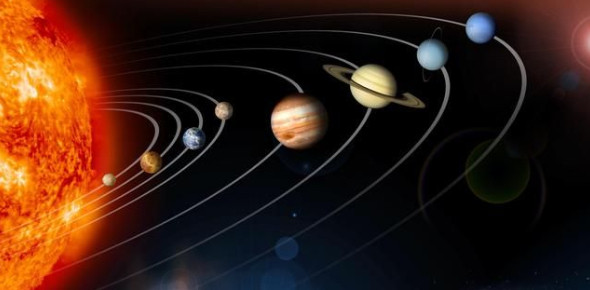# True Or False: Quiz On Solar System!

10 Questions | Total Attempts: 1845Settings.

• 1.
The asteroid is made of water and ice that moves around the Sun.
• A.

True

• B.

False

• 2.
Earth is the third planet in the Solar System.
• A.

True

• B.

False

• 3.
There are nine planets in the Solar System.
• A.

True

• B.

False

• 4.
A star is an object in space that gives out light and heat.
• A.

True

• B.

False

• 5.
Mercury is the smallest planet in the Solar System with a diameter of 3 000 km.
• A.

True

• B.

False

• 6.
Jupiter is 58 million km from the Sun.
• A.

True

• B.

False

• 7.
The Moon is an example of a natural satellite.
• A.

True

• B.

False

• 8.
Venus faces the Sun and has a very high temperature of 430 oC.
• A.

True

• B.

False

• 9.
Mercury is the hottest planet with surface temperature of about 462 oC.
• A.

True

• B.

False

• 10.
The Earth has surrounded by a layer of gases known as the atmosphere.
• A.

True

• B.

False

Related TopicsBack to top